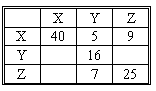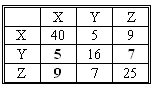### CFA Practice Question

There are 985 practice questions for this topic.

### CFA Practice Question

Consider the following covariance matrix for 3 different variables, X, Y, and Z:What are the values for Cov(Y,Z), Cov(Z,X), and Cov(Y,X)?

A. 9, 5, 7
B. 7, 9, 5
C. 4, 9, 7

Cov(Y,Z) = Cov(Z,Y) = 7, Cov(Z,X) = Cov(X,Z) = 9, and Cov(Y,X) = Cov(X,Y) = 5. Therefore, the complete correlation matrix looks like this: